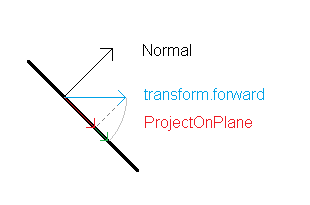# 3D Character Controller slowing down the higher the slope angle (both up and down)

Hi everyoneI am trying to make a 3D character controller but ran into an issue where my player would slow down going up slopes and would bounce going down. I have come up with a solution I was happy with but it seems to slow down the player any time they move up or down a slope. Basically, rather than driving my player towards transform.forward, I am getting the forward direction based on the current ground normal.

``````        forwardDir = Vector3.ProjectOnPlane(transform.forward, groundNormal);

if (rayGrounded)
{
targetSpeed = (((isRunning) ? runSpeed : walkSpeed) * inputDir.magnitude);
currentSpeed = Mathf.SmoothDamp(currentSpeed, targetSpeed, ref speedSmoothVelocity, GetModifiedSmoothTime(speedSmoothTime));
velocityY += Time.deltaTime * gravity;
velocity = ((forwardDir) * currentSpeed) + (Vector3.up * velocityY) + dashVelocity;
}

else
{
targetSpeed = (((isRunning) ? runSpeed : walkSpeed) * inputDir.magnitude);
currentSpeed = Mathf.SmoothDamp(currentSpeed, targetSpeed, ref speedSmoothVelocity, GetModifiedSmoothTime(speedSmoothTime));
velocityY += Time.deltaTime * gravity;
velocity = ((forwardDir) * currentSpeed) + (Vector3.up * velocityY) + dashVelocity;
}
playerController.Move((velocity * Time.deltaTime));
``````

Any help would be appreciated! This issue has been bugging me all day and im sure its a simple fix.

The method ProjectOnPlane does not rotate the given vector parallel to the plane but project it onto the plane:As you can see the steeper the angle the shorter the projected vector. Think about the shadow of a pole with light coming parallel from the normal direction. If the “pole” points in the same direction as the normal the shadow would completely disappear. If the pole is parallel to the plane the projected shadow will have the same length as the pole. What you get from ProjectOnPlane is the read arrow. What you probably want is the green arrow. The green arrow has the same length as the blue arrow.

All you have to do to get the green arrow is to normalize your projected vector.

``````forwardDir = Vector3.ProjectOnPlane(transform.forward, groundNormal).normalized;
``````